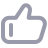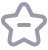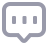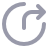# 【云和恩墨大讲堂】玩转AWR裸数据（下）

797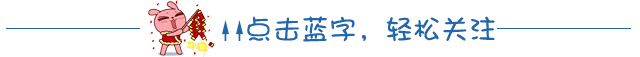编辑手记：云和恩墨大讲堂2017新年第一讲 - 玩转AWR裸数据，通过裸数据挖掘AWR更多的价值，有效帮助你进行数据库性能分析。ITPUB论坛数据库管理版版主，2012 ITPUB全国SQL大赛冠军得主，他还是资深的架构师和性能优化专家，对 SQL 优化和理解尤其深入；从开发到性能管理，他有着超过10年的企业级系统设计和优化经验。曾经服务于甲骨文公司，组织和主讲过多次《甲骨文技术开发人员日》和《Oracle圆桌会议》，并具备丰富的制造行业系统架构经验。

• value - lag(value) over(partition by stat_name order by snap_id) 可以获取两次snap_id间的数据区别

• 通过PIVOT, 可以轻松的做行列转行。

select * from (select snap_id,STAT_NAME,

value-lag(value) over(partition by STAT_NAME

order by snap_id) value

from dba_hist_sysstat where stat_name in (

) ) PIVOT (sum(value) for stat_name in (

))order by snap_id;

• 通过Ratio_To_Report() over() 可以获得当前行数据在所有同组数据内占的比例

select * from (select snap_id,STAT_NAME,

value-lag(value) over(partition by STAT_NAME

order by snap_id) value

from dba_hist_sysstat where stat_name in (

) ) PIVOT (sum(value) for stat_name in (

))order by snap_id;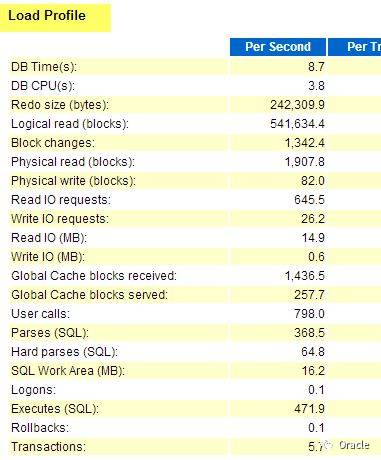• 第二个部分，是Top timed events, 最耗时间的等待事件（包括CPU)的部分。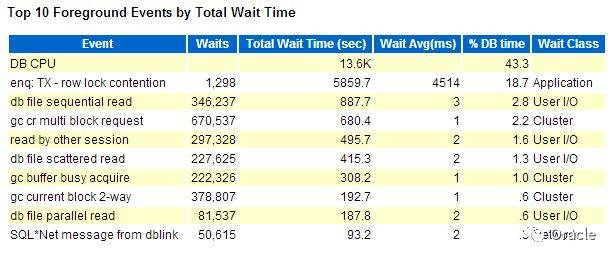select snap_id,event,pct||'%' PCT,time from (

select snap_id,event,round(time)time,

round(RATIO_TO_REPORT(TIME) over(partition by snap_id)*100,1) pct

from( select 'CPU Time' EVENT,snap_id,value/100 - LAG(value)over(partition by stat_name order by snap_id)/100 TIME

from DBA_HIST_SYSSTAT where stat_name = 'CPU used by this session'

union all select event_name,snap_id, time_waited_micro/1e6 -

LAG(time_waited_micro) over(partition by event_name order by snap_id)/1e6

from DBA_HIST_SYSTEM_EVENT where wait_class!='Idle'

)where time>0) where pct>1 order by snap_id,time desc

• 通常来说，知道了系统负载，系统瓶颈，我们还需要了解的是第三个部分： Top SQL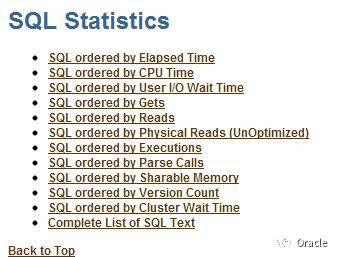select sql.*, (select SQL_TEXT from dba_hist_sqltext t

where t.sql_id = sql.sql_id and rownum=1 ) SQLTEXT

from (select a.* ,

RANK() over( order by els desc) as r_els,

RANK() over( order by phy desc) as r_phy,

RANK() over( order by get desc) as r_get,

RANK() over( order by exe desc) as r_exe,

RANK() over( order by CPU desc) as r_cpu

from (

select sql_id,sum(executions_delta) exe,round(sum(elapsed_time_delta ) 1e6, 2) els

,round(sum(cpu_time_delta) 1e6, 2) cpu,

round(sum(iowait_delta) 1e6, 2) iow,sum(buffer_gets_delta) get,

round(sum(elapsed_time_delta) greatest(sum(executions_delta), 1) 1e6,4) elsp,

round(sum(cpu_time_delta) greatest(sum(executions_delta), 1) 1e6, 4) cpup,

round(sum(iowait_delta) greatest(sum(executions_delta), 1) 1e6, 4) iowp,

round(sum(buffer_gets_delta) greatest(sum(executions_delta), 1), 2) getp,

round(sum(rows_processed_delta) greatest(sum(executions_delta), 1), 2) ROWP

from dba_hist_sqlstat s

--where snap_id between ... and ...

group by sql_id ) a

)SQL where r_els <= 10 or r_phy <=10 or r_cpu<=10 order by els desc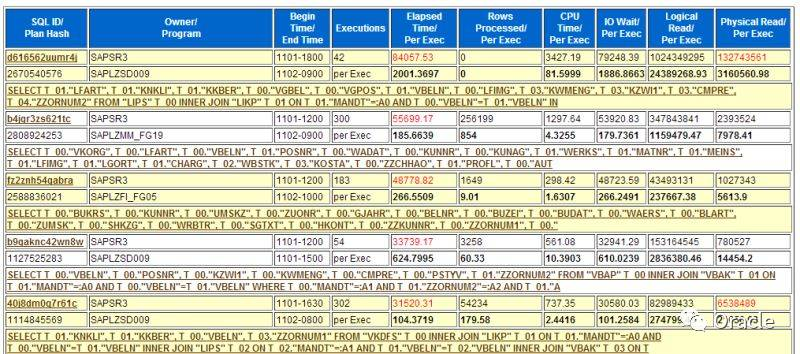select * from table(dbms_xplan.display_awr('&SQLID'))

DBA_HIST_SQLSTAT.BIND_DATA 这个栏位里面，保存了绑定变量

select snap_id,sq.sql_id,bm.position, dbms_sqltune.extract_bind(sq.bind_data,bm.position).value_string value_string

where sq.sql_id = bm.sql_id --and sq.sql_id = '&sql'

select * from ( select snap_id, to_char(sn.begin_interval_time,'MM/DD-HH24:MI') snap_time, sq.sql_id,bm.position, dbms_sqltune.extract_bind(bind_data,bm.position).value_string value_string from dba_hist_snapshot sn natural join dba_hist_sqlstat sq ,dba_hist_sql_bind_metadata bm

where sq.sql_id = bm.sql_id and sq.sql_id = '&sql'

) PIVOT (max(value_string) for position in (1,2,3,4,5,6,7,8,9,10))

order by snap_id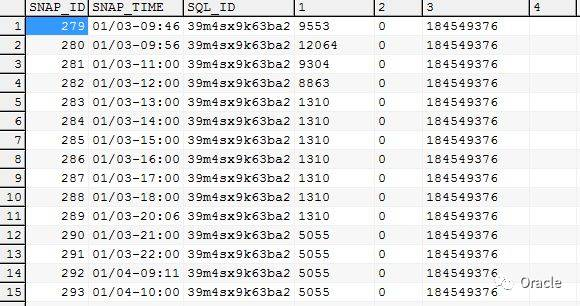Select plan_hash_value ,Sum(Elapsed_time_Delta) greatest(Sum(Executions_Delta),1),sum(Executions_Delta) From dba_hist_sqlstat where sql_id = '&SQLID' group by plan_hash_value ;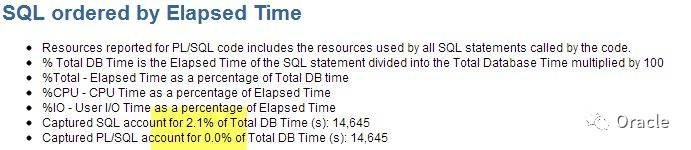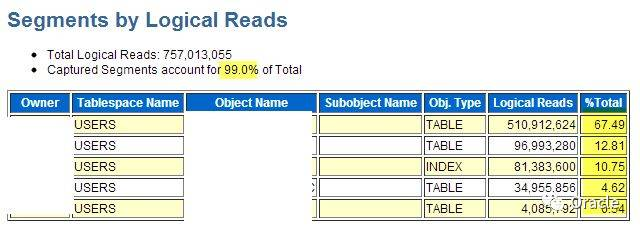from DBA_HIST_SEG_STAT SEG ,DBA_HIST_SEG_STAT_OBJ O , dba_hist_snapshot snap

where o.obj# = seg.obj# and o.dataobj# = seg.dataobj# and PHYSICAL_READS_DELTA > 1e5 and seg.snap_id = snap.snap_id

and begin_interval_time > sysdate - 4/24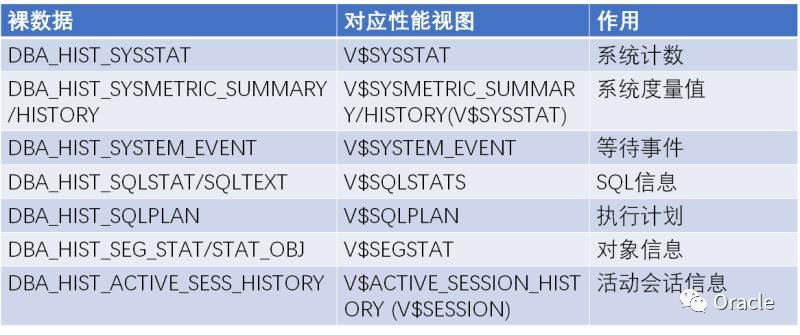AWR裸数据如此的重要，对于关心数据库性能的DBA们，我们需要好好的保护好它们~

1. 系统保存时间,默认7天远远不足，建议改到30天以上，跨过一个月结周期

2. 需要的时候，我们可以对裸数据进行离线备份

3. 甚至，我们可以把裸数据专门找个数据库存起来，作为一个资料库使用。

4. 有时候，也可以针对特定的表进行备份。比如说，我刚刚贴的这个列表

1. AWR的分析办法： Load Profile, Top Timed Event, Top SQL, SQL Plan, SQL 绑定变量,Top Segments

2. 一些高级SQL用法: 分析函数 Lag/Rank/Ratio_to_report, 行列转换 PIVOTLockdown Profile 的多租户权限控制

Oracle 12c Global Data Services

MySQL Group Replication 学习笔记